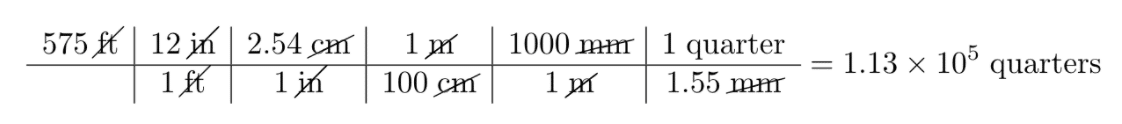# How Many Quarters Would Have To Be Stacked To Reach 575 Ft, The Height Of The Washington Monument?

We thoroughly check each answer to a question to provide you with the most correct answers. Found a mistake? Let us know about it through the REPORT button at the bottom of the page.

The U.S. quarter has a mass of 5.67 g and is approximately 1.55 mm thick. How many quarters would have to be stacked to reach 575 ft, the height of the Washington Monument?

## Explanation

1. Begin with the height in feet.
2. Use a conversion factor to convert feet into inches.
3. Use a conversion factor to convert inches into centimeters.
4. Use a conversion factor to convert centimeters into meters.
5. Use a conversion factor to convert meters into millimeters.
6. Use the thickness per quarter as a conversion factor to convert millimeters into the number of quarters.